# GROWTH Function

Calculates the predicted exponential growth by using existing data

## What is the GROWTH Function?

The GROWTH Function is categorized under Excel Statistical functions. The function helps calculate predicted exponential growth by using existing data.

In financial analysis, GROWTH helps in preparing annual plans or forecasting revenues for a company. Let’s assume the CEO expects annual revenues to reach \$1,500,000. The function can help us understand if the target is achievable or not by calculating the exponential growth.

### Formula

=GROWTH(known_y’s, [known_x’s], [new_x’s], [const])

The GROWTH function uses the following arguments:

1. Known_y’s (required argument) – This is the set of known y-values.
2. Known_x’s (optional argument) – This is the set of known x-values. If a user provides the argument, it should be of the same length as the set of known_y’s.
3. New_x’s (optional argument) – They are the new x_values for which we want GROWTH to return a corresponding value. If omitted, the set of [new_x’s] is assumed to be the same as the [known_x’s] and the function returns the y-values that lie on the calculated exponential growth curve.
4. Const (optional argument) – This is a logical value that deterines whether the constant ‘b’ in the equation y = b * m^x should be forced to be equal to the value 1.
• If const is TRUE or omitted, b is calculated normally.
• If const is FALSE, b is set equal to 1 and the m-values are adjusted so that y = m^x.

### How to use the GROWTH Function in Excel?

To understand the uses of the GROWTH function, let’s consider a few examples:

#### Example 1

Let’s assume we wish to know if sales of \$15 million are possible or not for the coming financial year. So:

• Known_y’s are the revenue values that we know.
• Known_x’s are the years for which revenue data are available.
• New_s’s are the years for which we want to predict the revenue.

Suppose we are given the following data: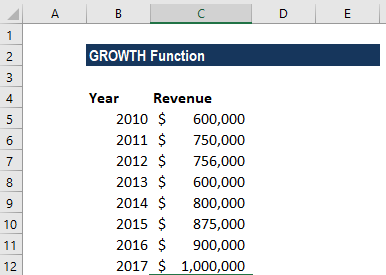In the above example, the known_y’s are the revenue figures in column C, whereas the known_x’s are the year information in column B. The new_x is B13.

The formula to use will be: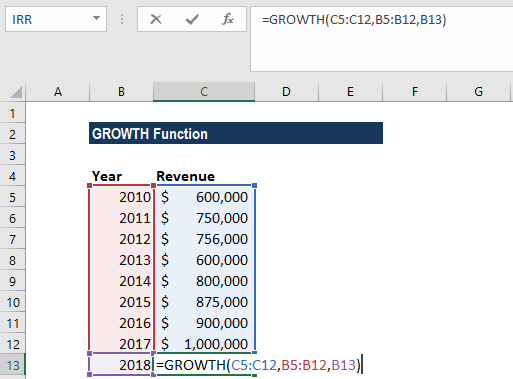Remember we are providing a cell reference, so no quotation marks required. We get the result below: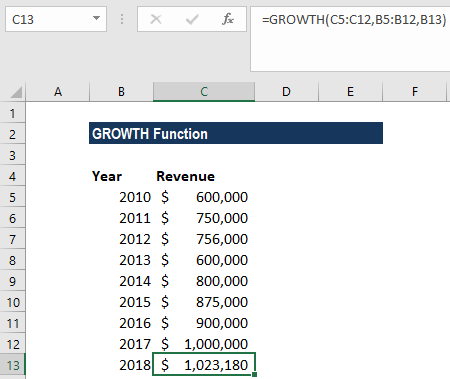From the result above, \$1,500,000 seems not possible. \$1,023,180 seems more achievable.

#### Example 2

Continuing with the same example, if we wish to find out the revenue that is more than one new y-value, we need to use the array formula. The function should be entered as an array formula.

For this, we will enter an array formula, then highlight the range of cells for the function result. First, we will type the function into the first cell of range and then press CTRL-SHIFT-Enter, as shown below: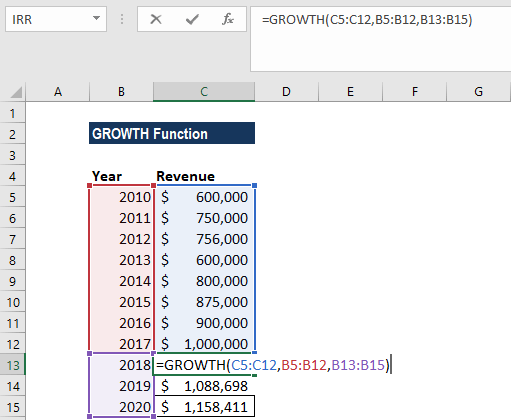We get the result below: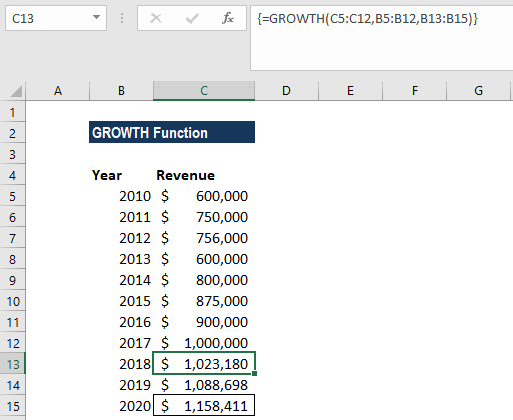As you can see above, the GROWTH function was entered into cells C13-C15 and the function in the formula bar is encased in curly braces { }. It indicates that the function was entered as an array formula.

### A few notes about the GROWTH function

1. #REF! error – Occurs if the [known_x’s] array is of a different length compared to the known_y’s array.
2. #NUM! error – Occurs if any of the values in the known_y’s array is less than or equal to zero.
3. #VALUE! error – This occurs if any of the values provided is non-numeric for known_y’s, [known_x’s] or [new_x’s] arrays.
4. If we wish to see the GROWTH function visually, we can create a scatter chart of the data without displaying a line. The exponential option uses the function while the linear option uses the TREND function.

Thanks for reading CFI’s guide to important Excel functions! By taking the time to learn and master these functions, you’ll significantly speed up your financial analysis. To learn more, check out these additional CFI resources:

• Excel Functions for Finance